## Saturday, April 12, 2014

### 3. Reading a Wave File

Now we will see how Python reads a wave file. All computer files are binary data, that is made of 0's and 1's. However we always deal with bytes, which are the smallest addressable group and they are 8-bits in length. A byte can hold 256 values, which is 2 to the power of 8.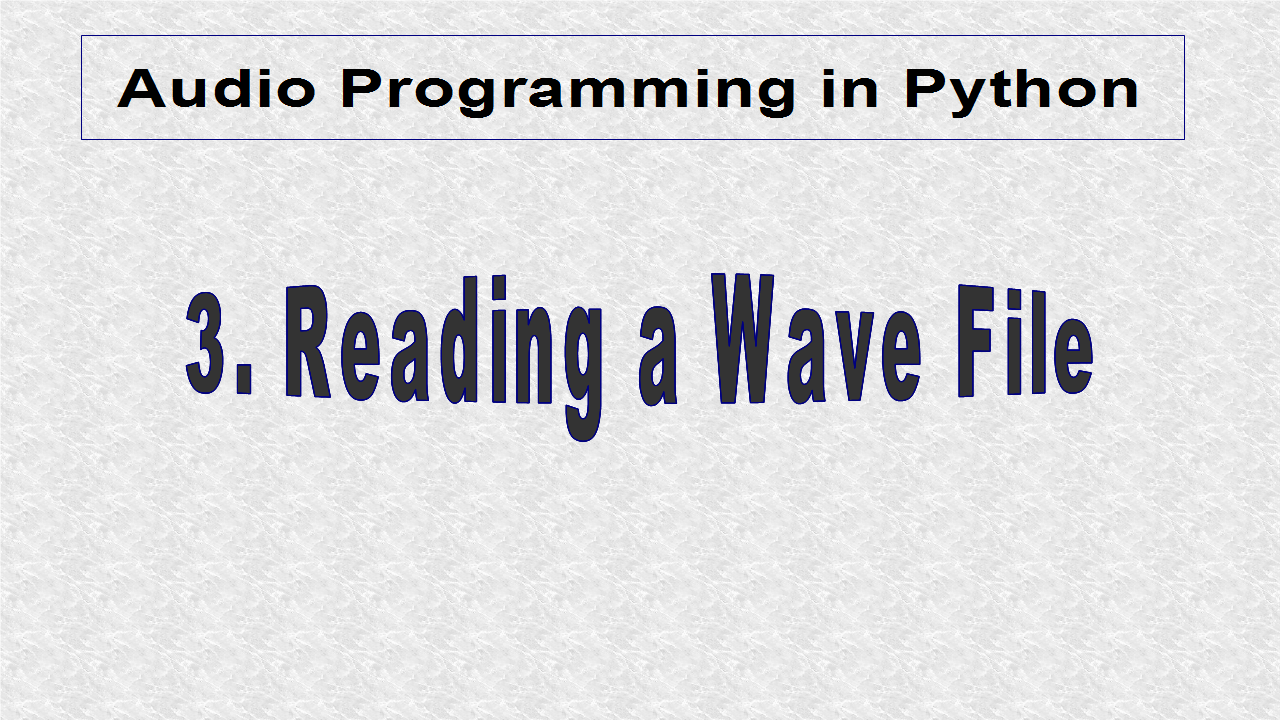A stereo wave file consists of 2 channels; left and right. Each channel sample is of 16-bit length. A 16-bit number can represent 65,536 values, 2 to the power of 16. A signed 16-bit integer can represent anything from -32,768 to +32,767.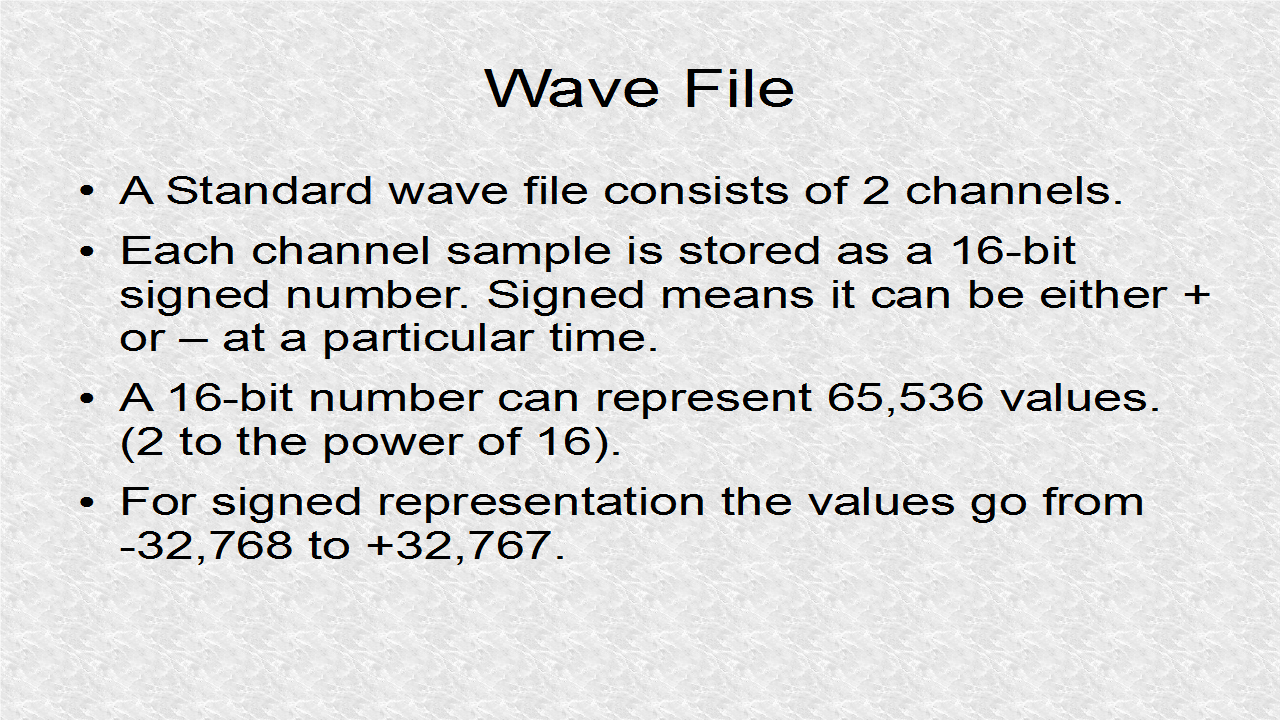Now we will calculate the size of a 1-minute mono wave file. In a mono wave file, there are 44100 samples per second. This rate is based on the Nyquist Theorem which states that to retain the frequency f, we should sample at a rate of 2f. Thus 44100 sample rate, should be able to reconstruct audio data for frequencies until 22,050 Hz. There are 44100 samples per second, and since each sample is 2 bytes, we have 88,200 Bytes/second. If we multiply this number by 60 seconds, we get 5,292,000 Bytes. The actual wave file size is 5,292,044 (we always have to include a 44-byte header). The header will have some identifying information and also information about the kind of audio signal.Likewise the DataSize for a 1-minute stereo file is double the number above, and we have 10,584,000 Bytes. Again the actual size of the wave file is 10,584,044 Bytes, resulting from the 44-byte header.Most audio is not stored as wave, but compressed formats to save disk space. However to do data analysis we need the wave format. Likewise after data synthesis, we will write to the wave format.As mentioned the wave file has 44 byte header. The information in the header is thoroughly documented on the internet and you could search for the specs. Now two methods of reading wave files are discussed. The first method is important to understand how Python deals with files. It is also helping in understanding the structure of pysynth file. The second method is the recommended way and is more abstract in that it hides the details. Furthermore it is more efficient.To read a file in Python we have to use the open command. The open command needs two arguments. One is the filename, and other is mode. The two modes for wave files are 'rb' and 'wb' for reading and writing binary files. Whenever you read a Byte in Python, a string is returned from '\0x00' to '\0xff'. \0x is the standard way of declaring something is hexadecimal in Python (the hex values are 0 to 9, and a to f (the letters can be either lowercase or capital.This is an example of a string that might be returned by reading the binary file. We can see the string contains 4 hex digits (2 bytes or 16 bits). We can decode this to a number between -32,768 and +32,767.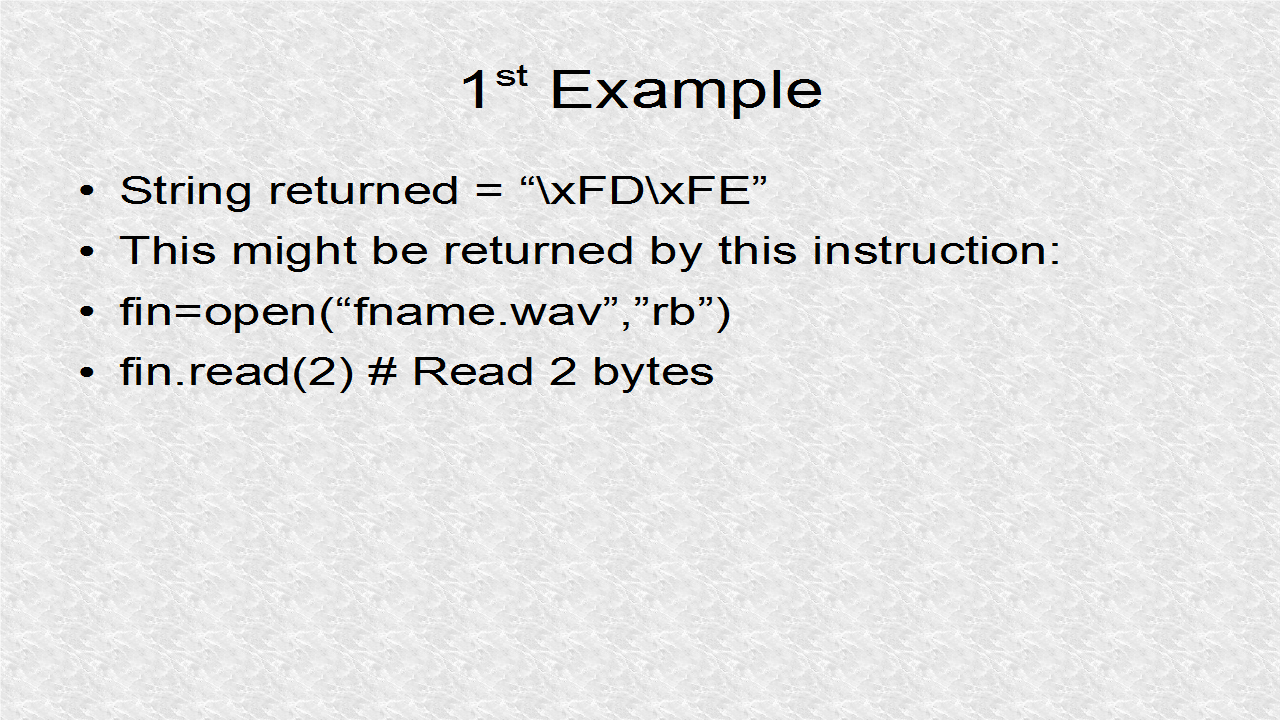The string is read as FEFD. This is called the little endian format. Basically, in less technical terms, it means that we reverse the string bytes. In the string FD comes first, and FE is second however we read it as the reverse for the conversions. Most computers are of little endian format. FEFD can be represented as a 16-bit binary number. Since it starts with 1 (leftmost bit) the number is negative – the first bit is always the sign bit in signed representations. The absolute value can be obtained by the 2's complement, inverting all bits and then adding 1. The 2's complement gives an absolute value of 259. Thus the number is actually -259.Rather than deal with all this math, we can let the computer figure it. We input the string to an unpack instruction. We have to tell the unpack function we want it to treat the string as signed 16-bit integer, which is the 'h' flag. All the different flags can be found in the documentation. The unpack function in struct module results in a tuple – which can be thought as a set of numbers. Our set is just one number. We will go over tuple and other data structures in detail later. Then I extract the 0'th element (which is the only element. I write underscore 3 to get Out variable.In the second example, a positive number is in the binary string. The hex is 1405, which is just the reverse of the string. If 1405 hex is written as binary, we can see the leftmost bit is 0, and thus the number is positive, and value is 5125.We can verify, with Python code, that indeed 5125 is the value of string.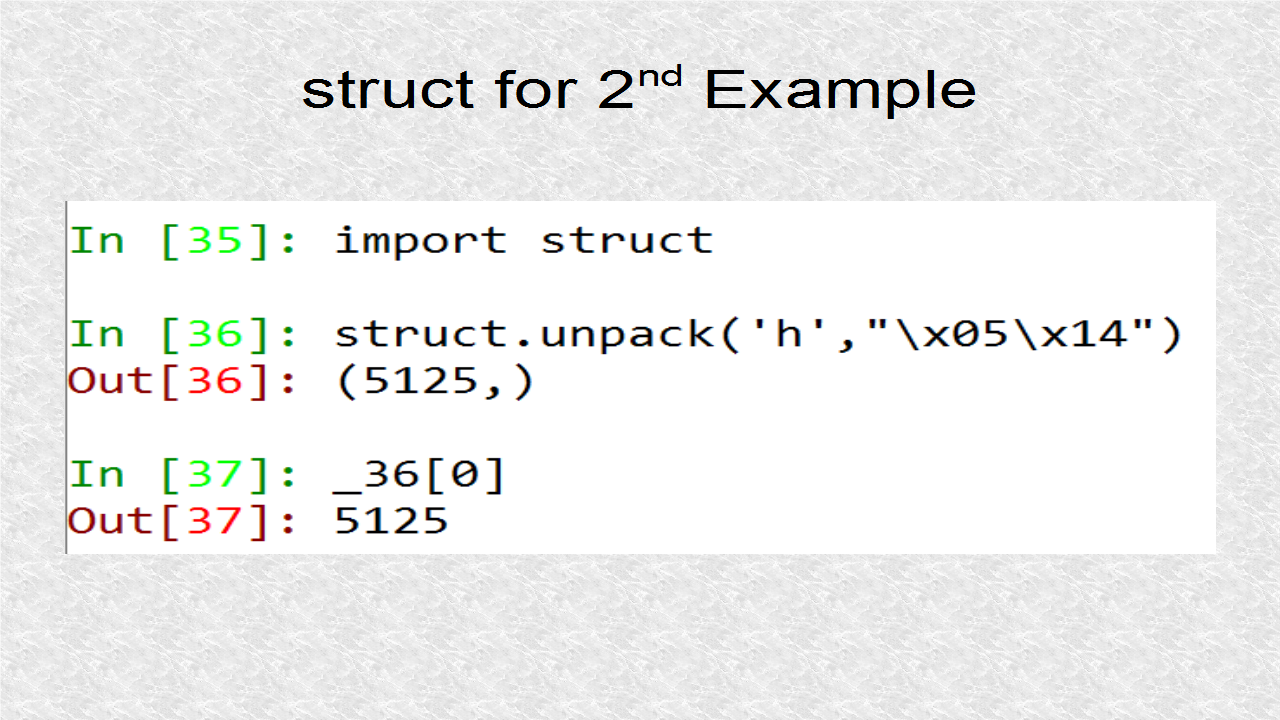I have a test file called waverd.py which I will upload to pythonaudio.blogspot.com. To understand this file, we have to understand how we can read a 2-byte number or 4-byte number using the read instruction. The data is signed. However everything else is treated as unsigned and the format flags are H (for 2 byte integers) and I (for 4 byte integer). A lot of headers are ASCII text codes, and unpacking is not necessary for them, as print can easily deal with them.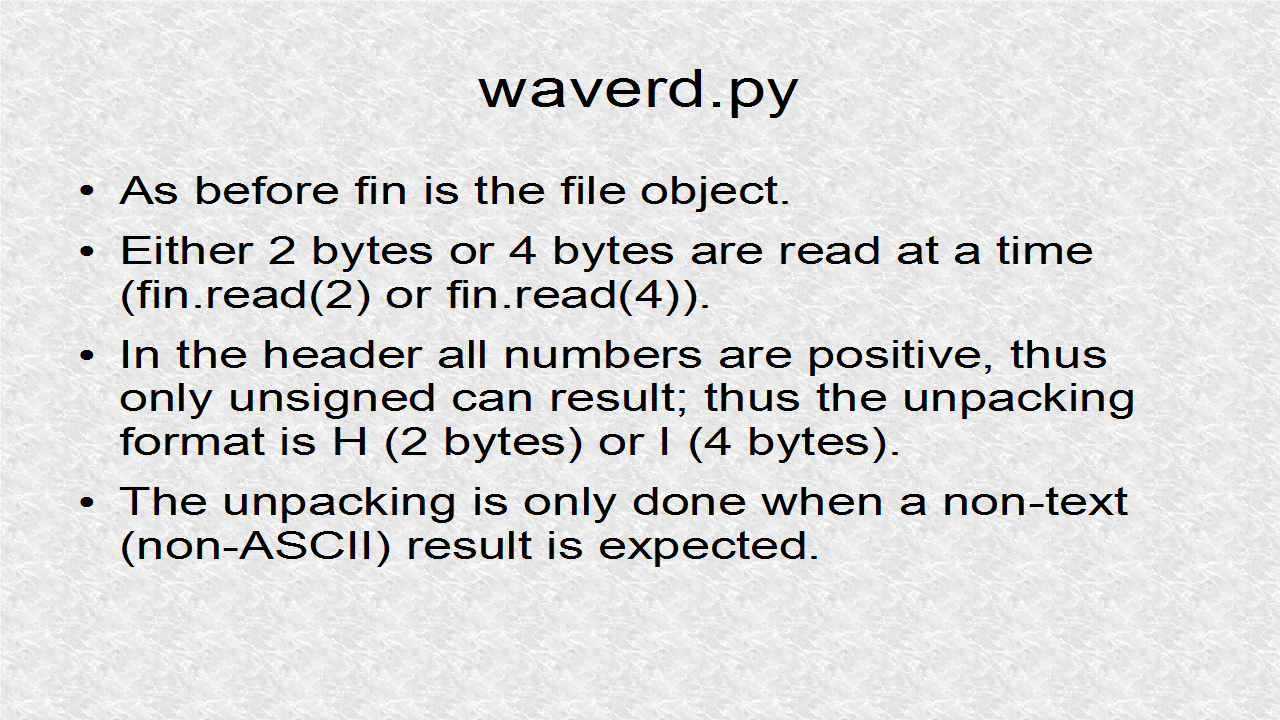```# waverd.py
# When reading a binary file, Python converts values to strings.
# To decode the strings we need the struct module

import struct
# open(fname,mode) is the Python way of reading files
fin = open("pysynth_scale.wav","rb") # Read wav file, "r flag" - read, "b flag" - binary
ChunkID=fin.read(4) # First four bytes are ChunkID which must be "RIFF" in ASCII
print("ChunkID=",ChunkID)
ChunkSizeString=fin.read(4) # Total Size of File in Bytes - 8 Bytes
ChunkSize=struct.unpack('I',ChunkSizeString) # 'I' Format is to to treat the 4 bytes as unsigned 32-bit inter
TotalSize=ChunkSize+8 # The subscript is used because struct unpack returns everything as tuple
print("TotalSize=",TotalSize)
DataSize=TotalSize-44 # This is the number of bytes of data
print("DataSize=",DataSize)
print("Format=",Format)
SubChunk1ID=fin.read(4) # "fmt " in ASCII
print("SubChunk1ID=",SubChunk1ID)
SubChunk1SizeString=fin.read(4) # Should be 16 (PCM, Pulse Code Modulation)
SubChunk1Size=struct.unpack("I",SubChunk1SizeString) # 'I' format to treat as unsigned 32-bit integer
print("SubChunk1Size=",SubChunk1Size)
AudioFormatString=fin.read(2) # Should be 1 (PCM)
AudioFormat=struct.unpack("H",AudioFormatString) # 'H' format to treat as unsigned 16-bit integer
print("AudioFormat=",AudioFormat)
NumChannelsString=fin.read(2) # Should be 1 for mono, 2 for stereo
NumChannels=struct.unpack("H",NumChannelsString) # 'H' unsigned 16-bit integer
print("NumChannels=",NumChannels)
SampleRateString=fin.read(4) # Should be 44100 (CD sampling rate)
SampleRate=struct.unpack("I",SampleRateString)
print("SampleRate=",SampleRate)
ByteRateString=fin.read(4) # 44100*NumChan*2 (88200 - Mono, 176400 - Stereo)
ByteRate=struct.unpack("I",ByteRateString) # 'I' unsigned 32 bit integer
print("ByteRate=",ByteRate)
BlockAlignString=fin.read(2) # NumChan*2 (2 - Mono, 4 - Stereo)
BlockAlign=struct.unpack("H",BlockAlignString) # 'H' unsigned 16-bit integer
print("BlockAlign=",BlockAlign)
BitsPerSampleString=fin.read(2) # 16 (CD has 16-bits per sample for each channel)
BitsPerSample=struct.unpack("H",BitsPerSampleString) # 'H' unsigned 16-bit integer
print("BitsPerSample=",BitsPerSample)
print("SubChunk2ID=",SubChunk2ID)
SubChunk2SizeString=fin.read(4) # Number of Data Bytes, Same as DataSize
SubChunk2Size=struct.unpack("I",SubChunk2SizeString)
print("SubChunk2Size=",SubChunk2Size)
S1=struct.unpack("h",S1String)
print("S1=",S1)
S2=struct.unpack("h",S2String)
print("S2=",S2)
S3=struct.unpack("h",S3String)
print("S3=",S3)
S4=struct.unpack("h",S4String)
print("S4=",S4)
S5=struct.unpack("h",S5String)
print("S5=",S5)
fin.close()
```

This is the output of waverd.py file. It reads the header and all values are consistent with a mono signal. It also reads the first 5 samples. If we were to read a stereo file each sample would correspond to 4 bytes, 2 bytes for left and 2 bytes for right channel. Now the correct formatting flag is 'hh' to indicate two separate 2-byte signed numbers.From the Header we can find the DataSize and also the ByteRate. By dividing the two numbers we can find how many seconds are in the wav file.```# waverd_1.py
# When reading a binary file, Python converts values to strings.
# To decode the strings we need the struct module

import struct
# open(fname,mode) is the Python way of reading files
fin = open("pysynth_scale.wav","rb") # Read wav file, "r flag" - read, "b flag" - binary
ChunkID=fin.read(4) # First four bytes are ChunkID which must be "RIFF" in ASCII
#print("ChunkID=",ChunkID)
ChunkSizeString=fin.read(4) # Total Size of File in Bytes - 8 Bytes
ChunkSize=struct.unpack('I',ChunkSizeString) # 'I' Format is to to treat the 4 bytes as unsigned 32-bit inter
TotalSize=ChunkSize+8 # The subscript is used because struct unpack returns everything as tuple
#print("TotalSize=",TotalSize)
DataSize=TotalSize-44 # This is the number of bytes of data
print("DataSize=",DataSize)
#print("Format=",Format)
SubChunk1ID=fin.read(4) # "fmt " in ASCII
#print("SubChunk1ID=",SubChunk1ID)
SubChunk1SizeString=fin.read(4) # Should be 16 (PCM, Pulse Code Modulation)
SubChunk1Size=struct.unpack("I",SubChunk1SizeString) # 'I' format to treat as unsigned 32-bit integer
#print("SubChunk1Size=",SubChunk1Size)
AudioFormatString=fin.read(2) # Should be 1 (PCM)
AudioFormat=struct.unpack("H",AudioFormatString) # 'H' format to treat as unsigned 16-bit integer
#print("AudioFormat=",AudioFormat)
NumChannelsString=fin.read(2) # Should be 1 for mono, 2 for stereo
NumChannels=struct.unpack("H",NumChannelsString) # 'H' unsigned 16-bit integer
#print("NumChannels=",NumChannels)
SampleRateString=fin.read(4) # Should be 44100 (CD sampling rate)
SampleRate=struct.unpack("I",SampleRateString)
#print("SampleRate=",SampleRate)
ByteRateString=fin.read(4) # 44100*NumChan*2 (88200 - Mono, 176400 - Stereo)
ByteRate=struct.unpack("I",ByteRateString) # 'I' unsigned 32 bit integer
print("ByteRate=",ByteRate)
BlockAlignString=fin.read(2) # NumChan*2 (2 - Mono, 4 - Stereo)
BlockAlign=struct.unpack("H",BlockAlignString) # 'H' unsigned 16-bit integer
#print("BlockAlign=",BlockAlign)
BitsPerSampleString=fin.read(2) # 16 (CD has 16-bits per sample for each channel)
BitsPerSample=struct.unpack("H",BitsPerSampleString) # 'H' unsigned 16-bit integer
#print("BitsPerSample=",BitsPerSample)
#print("SubChunk2ID=",SubChunk2ID)
SubChunk2SizeString=fin.read(4) # Number of Data Bytes, Same as DataSize
SubChunk2Size=struct.unpack("I",SubChunk2SizeString)
#print("SubChunk2Size=",SubChunk2Size)
S1=struct.unpack("h",S1String)
#print("S1=",S1)
S2=struct.unpack("h",S2String)
#print("S2=",S2)
S3=struct.unpack("h",S3String)
#print("S3=",S3)
S4=struct.unpack("h",S4String)
#print("S4=",S4)
S5=struct.unpack("h",S5String)
#print("S5=",S5)
fin.close()
```

This is the other way of reading a standard wav file. It imports io.wavfile submodule from the scipy module. By using the read function inside this submodule, we can read a binary file. This is more abstract, and is more efficient. Next I extract five elements from the data using slicing.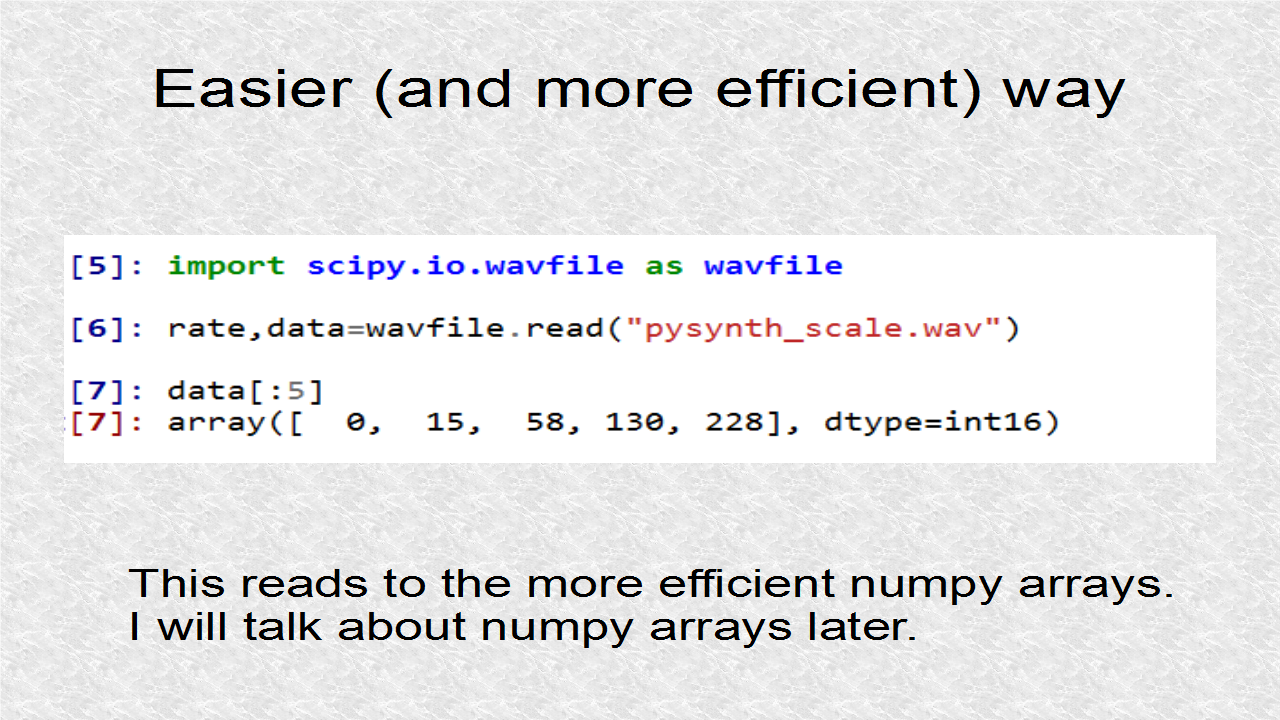The reason I spent so much time on details of struct, is so it easier to understand PySynth which uses that function. It also uses another module, called wave, which makes reading wav files easier than using Python open command. This hides more details of the header as can be seen next.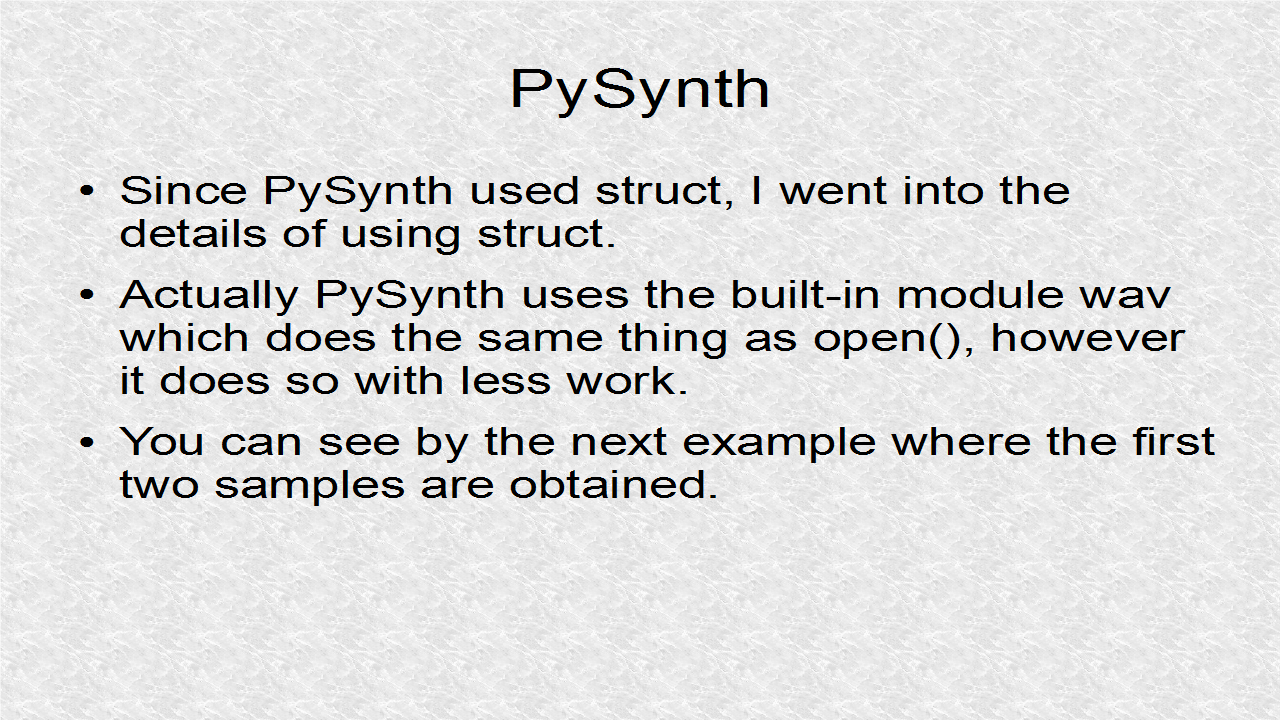The struct module is used to read a few data values. I show the commands to read the first 2 samples.```# waverdwave.py
import struct
import wave
wrd=wave.open("pysynth_scale.wav","r")
S1=struct.unpack('h',S1String)
print("S1=",S1)
S2=struct.unpack('h',S2String)
print("S2=",S2)
S3=struct.unpack('h',S3String)
print("S3=",S3)
S4=struct.unpack('h',S4String)
print("S4=",S4)
S5=struct.unpack('h',S5String)
print("S5=",S5)
```

This shows the first 5 samples.In PySynth, most of the time is spent on writing wave files. To understand writing of wave files, it helps understanding how wave files are read, and then using the set and write functions in documentation, we can write a wave file.This is how you get documentation on the wave module which will give more information about the set commands.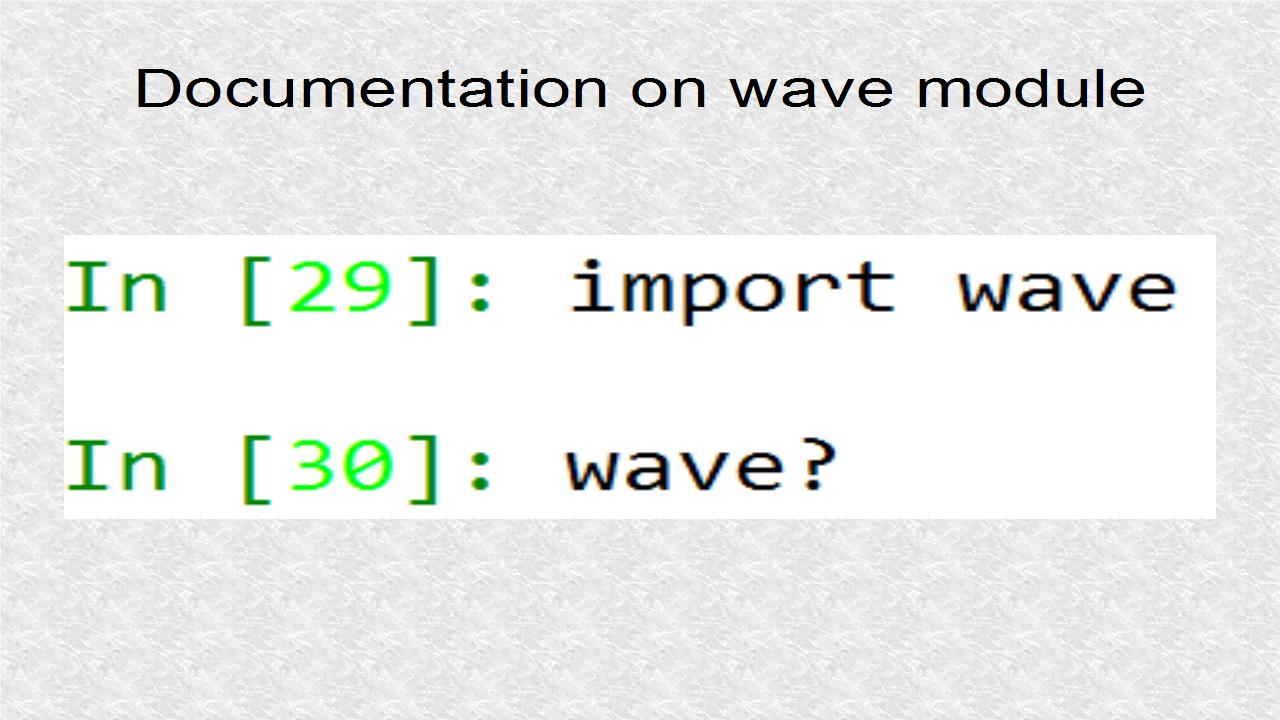You may go to pythonaudio.blogspot.com to see the slides. To see a larger image of the slide, you can click on them at that page, which provides easy navigation controls. The text for the audio of the slides as well as any relevant source code is also on that page.

This is the video of Tutorial 3:

1.Your Blog is very useful for me thanks.

2.I like your blog, I read this blog please update more content on python, further check it once at python online training

3.This is pretty much blog.

4.5.Thanks a ton for your explanation!!

6.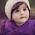This is an amazing blog, thank you so much for sharing such valuable information with us
7.8.